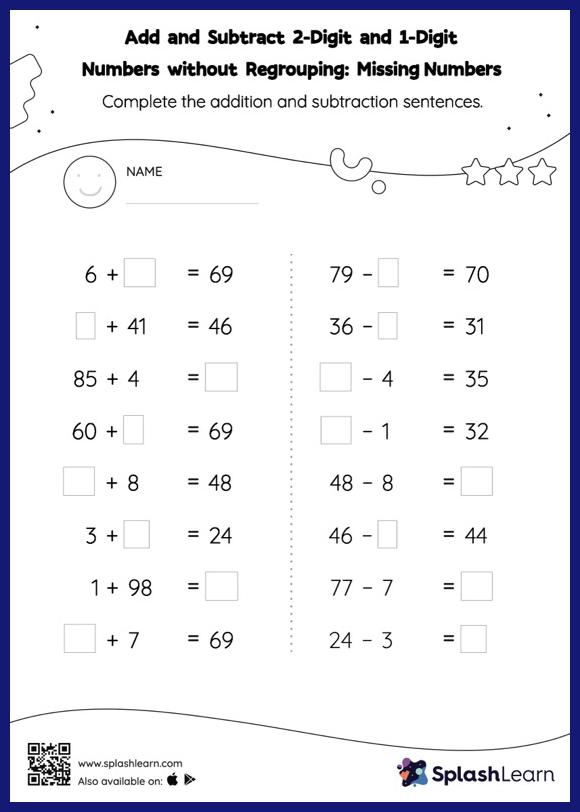# Add and Subtract 2-Digit and 1-Digit Numbers without Regrouping: Missing Numbers Worksheet

Home > Add and Subtract 2-Digit and 1-Digit Numbers without Regrouping: Missing NumbersIn this worksheet, students must find the missing number using the relationship between addition and subtraction. To solve add and subtract 2-digit and 1-digit numbers without regrouping worksheet, they do not need to regroup the numbers. As the worksheet uses a horizontal format, it allows for more creativity when it comes to how the student solves the problem. In contrast, in the vertical format, students usually employ the standard method to solve.